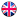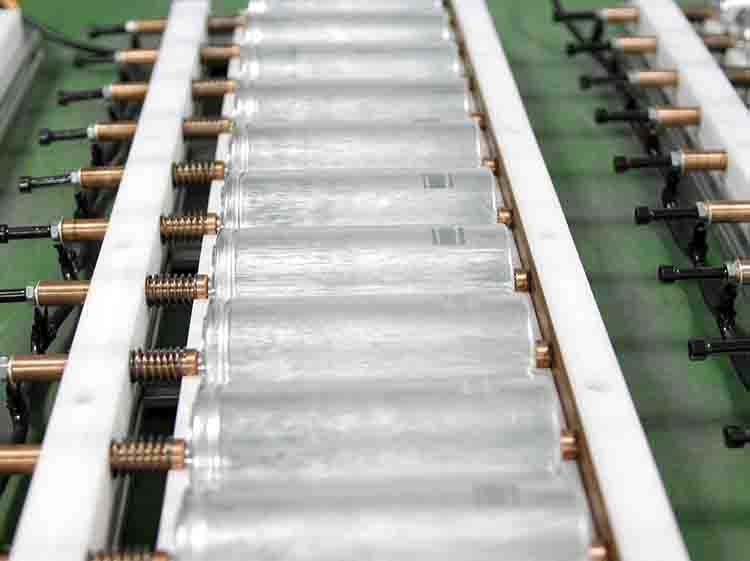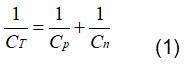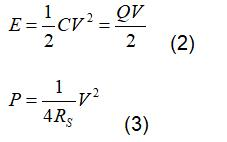EnglishHot keywords :

2019-11-15

#1.1Specific Capacitance

In super capacitor, each electrode is a capacitor, so for the super capacitor, the total capacitance is obtained by two capacitors in series. If the positive capacitance is represented by Cp and the negative capacitance by Cn, the total capacitance is:When doing test, researchers are more concerned about the specific capacitance of super capacitor, which is divided into two types: one is the specific capacitance of mass, that is, the capacitance value of unit mass. the other is the specific capacitance of volume, that is, the capacitance value of unit volume.

1.2 Energy Density And Power Density

Energy density and power density are the main standards to characterize the performance of super capacitors. The higher the energy density means the more energy the super capacitors can store. The higher the power density means the more energy the super capacitors can release in unit time. Generally speaking, the theoretical maximum energy density E and power density P of an ideal supercapacitor can be calculated by the following two formulas:In above formulas, C represents specific capacitance. V represents potential window, that is, the difference between the positive oxygen evolution potential and the negative hydrogen evolution potential of the electrolyte. RS is the equivalent series resistance of the capacitor. Q represents total charge stored in the capacitor. It released from formula (3) that the energy density of the super capacitor is directly proportional to the square of the specific capacitance and the potential window, the specific capacitance is related to the electrode material, and the potential window is related to the electrolyte The energy density and power density of the supercapacitor are directly proportional to the square of the potential window, so it is more effective to expand the potential window to improve the performance of the supercapacitor than to increase the capacitance value.

1.3 Internal Resistance

The internal resistance of the supercapacitor refers to the series resistance between the positive and negative capacitors, which is closely related to the electrode material, electrolyte, diaphragm and assembly mode. Smaller internal resistance is typically beneficial to the performance improvement of super capacitor

The greater the electrode thickness is, the higher the internal resistance is. The electrode common thickness should be less than 150μm. Moreover, the pore diameter of the material should not be too small, more than 1.5nm in typical. So as to facilitate the ions in the electrolyte to enter and fully infiltrate in order to form a double electric layer and avoid the internal resistance increase.

The resistance of electrolyte is also the main factor that affects the internal resistance of super capacitor. For water electrolyte, the diameter of ion is very small, the mobility is high, and it can easily pass through the diaphragm, and it can easily enter into the pores of electrode, so the internal resistance is very small. For organic electrolyte, the solute is generally organic polymer, the diameter is very large, and the probability of being blocked in the migration process is large It is much more difficult to enter the pores of the electrode, and the internal resistance is relatively higher.

1.4Cycling Stability

Cycling stability refers to the ability of supercapacitors to maintain electrical performance after multiple charging and discharging, which is mainly reflected in whether the attenuation degree of capacitance value is too large after multiple charging and discharging .The cycle stability is calibrated by the attenuation degree of the capacitance value after thousands of cycles of charging and discharging of super capacitor. The capacitance value measured in the first cycle is the initial value, and the capacitance value of the device after thousands of cycles of charging and discharging is the final value. The curve of the capacitance of super capacitor changing with the times of charging and discharging can be drawn by recording the capacitance value every hundreds of times between the initial value and the final value. Comparing the initial value with the final value, we can get the capacitor retention rate of the supercapacitor. Due to the erosion of the electrolyte to the electrode materials, especially the Faraday pseudocapacitor, the capacitance of the supercapacitor has a certain attenuation. However, the cycle life of super capacitor is still far ahead of that of battery, which is also a big advantage of super capacitor.